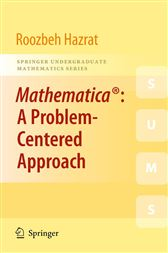# Mathematica®: A Problem-Centered Approach

## Series: Springer Undergraduate Mathematics Series (No. 53)36,99 €
###### ISBNs
• 1849962510
• 9781849962506
• 9781849962513

Mathematica®: A Problem-Centered Approach introduces the vast array of features and powerful mathematical functions of Mathematica using a multitude of clearly presented examples and worked- out problems. Each section starts with a description of a new topic and some basic examples. The author then demonstrates the use of new commands through three categories of problems

- the first category highlights those essential parts of the text that demonstrate the use of new commands in Mathematica whilst solving each problem presented;

- the second comprises problems that further demonstrate the use of commands previously introduced to tackle different situations; and

- the third presents more challenging problems for further study.

The intention is to enable the reader to learn from the codes, thus avoiding long and exhausting explanations.

While based on a computer algebra course taught to undergraduate students of mathematics, science, engineering and finance, the book also includes chapters on calculus and solving equations, and graphics, thus covering all the basic topics in Mathematica. With its strong focus upon programming and problem solving, and an emphasis on using numerical problems that do not need any particular background in mathematics, this book is also ideal for self-study and as an introduction to researchers who wish to use Mathematica as a computational tool.

Mathematica®: A Problem-Centered Approach comes with a free 30 day trial of the Wolfram Mathematica(R) software'

• Springer London; June 2010
• ISBN: 9781849962513
• Edition: 1
• Title: Mathematica®: A Problem-Centered Approach
• Series: Springer Undergraduate Mathematics Series (No. 53)
• Author: Roozbeh Hazrat
• Imprint: Springer
###### ISBNs
• 1849962510
• 9781849962506
• 9781849962513

#### In The Press

From the reviews:

“This is a pleasant and concise introduction to Mathematica, concentrating on its data structures and control structures … . I say the book is ‘pleasant’ because reading it is like having the author sitting next to you, answering questions just as you raise them. … valuable book, despite its simplicity and introductory nature. Even though I’ve been using Mathematica for about fifteen years, I still learned several useful things from the book.” (Allen Stenger, The Mathematical Association of America, December, 2010)

“Mathematica is a powerful commercial computer algebra program. … will allow users to solve a wide range of mathematical, science, engineering, economics, and other problems. Here, Hazrat … uses a selection of problems to instruct the reader in the basic capabilities of Mathematica and its programming language. … This volume will be most useful for libraries at institutions that have arranged institutional licenses to Mathematica. Summing Up: Recommended. Academic, two-year technical program, and professional libraries, all levels.” (D. Z. Spicer, Choice, Vol. 48 (6), February, 2011)

“Dr. Hazrat’s book aims to introduce and teach Mathematica to both beginners and those looking to understand some of the finer details of programming in Mathematica. … is much better suited to a course in number theory or programming. … contains many short Mathematica programs that solve simple mathematical problems. The programs demonstrate how to use a wide range of Mathematica commands to solve a wide array of problems. … I enjoyed reading this book and learning a number of Mathematica ‘tricks’ while doing so.” (John D. Carter, SIAM Review, Vol. 53 (3), 2011)

“This book is an elaborate hands-on tutorial for Mathematica … . Exercises are given at the end of each section. For each problem and exercise a solution is given. … The book is intended for self-study. Students with no particular background in mathematics as well as researchers in search of a computational tool should be able to delve quickly into the world of symbolic computation offered by Mathematica.” (Rainer Horsch, Zentralblatt MATH, Vol. 1211, 2011)

###### ISBNs
• 1849962510
• 9781849962506
• 9781849962513